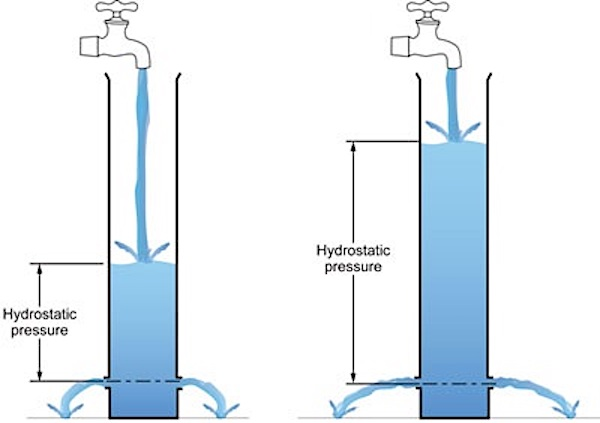Technical Article

# Alternate Uses for Differential Pressure Measurements

June 22, 2021 by Seth Price

## Besides measuring pressure to compare different parts of a process, differential pressure transmitters can also measure fluid level, volume, and flow.

Besides measuring the pressure of a fluid, pressure gauges can be used to determine several other physical quantities. In industry, pressure gauges can be used to determine the level of liquid in a tank or calculate the flow of a fluid through a pipe.

### Level Gauge or Volume Gauge

One method of determining the level of fluid in a tank is to use a differential pressure gauge. Typically, this is done by placing one end of the pressure sensor at the bottom of a tank and the other end exposed to the open atmosphere. It basically measures the gauge pressure of the bottom of the tank.

The pressure applied by the bottom of the tank is also called hydrostatic pressure. Hydrostatic pressure is governed by the equation below:

Phydrostatic = ρ * g * h

where ρ is the density of the liquid, g is the gravitational acceleration constant (9.81 m/s2 or 32.17 ft/s2 on Earth), and h is the height of the liquid in the tank. Notice that volume does not enter this equation at all—the hydrostatic pressure is the same at the bottom of a thin, deep tank as it is a very large tank with the same height.##### Figure 1. Hydrostatic pressure increases with depth. Image used courtesy of Mini Physics

With a standard 4-20 mA sensor, an engineer could calibrate the signal to correspond to an absolute height (in feet or meters), or in a “percent full,” where a signal of 4 mA means the tank is empty, and a signal of 20 mA means the tank is full. The advantage to “percent full” is that a technician can quickly glance at a display and understand “30% full” versus “7 meters.”

Depending on the application, the “percent full” measurement may be more useful than a volumetric measurement. If there is the concern of overflowing a tank, it is also much easier to understand “30% full” versus “358 gallons,” where the technician has to recall or research the size of that particular tank.

However, for some applications, such as batching and mixing, the volume may be more useful. The same standard 4-20 mA sensor can be calibrated for a volumetric measurement, provided the technician knows (or can find) the tank’s dimensions.

### Flow Meter

All fluid flows from higher pressure areas to lower pressure areas. Anyone who has ever played with a squirt gun, released the end of an untied balloon, or left a plastic bottle in a hot car has observed this effect.##### Figure 2. A critical orifice plate. The inner diameter of this orifice must be smaller than the pipe diameter.

Differential pressure measurements can determine how much fluid flows by taking advantage of this same effect. A differential pressure measurement can be placed along a pipe. A plate with a small hole, called a “critical orifice plate,” is placed between the two sensing elements. The higher the flow through the pipe, the larger the pressure drop will be across the critical orifice plate.

In fluid dynamics, the Bernoulli Equation and the Venturi Effect are used to relate fluid speed, pressure, nature of flow (laminar versus turbulent), and the size of the pipes (and critical orifice plates). A full explanation of these equations is beyond the scope of this article.

The key to good flow meter measurements is to verify the critical orifice diameter and ensure that it is the smallest diameter (biggest restriction) in the flow path. While the flow can be calculated from the fluid dynamics equations, sometimes, it is difficult to find all of the parameters needed for these equations.

Instead, the flow rate can always be calculated against a trusted flow meter, or, for non-hazardous liquids, a simple “bucket” test, where fluid is allowed to flow into a bucket and weighed for a specified amount of time to find a mass flow rate.

Differential pressure gauges are commonly used in industry. Besides measuring pressure to compare different parts of a process, they can also measure fluid level, volume, and flow, depending on their configuration. As it turns out, most gauge pressure sensors are technically differential pressure gauges, but one side is exposed to atmospheric pressure rather than another part of the process.

1 Comment• G
garyhlucas June 26, 2021

When I clicked on this article I was expecting to read about a differential pressure sensor.  Differential pressure measurements using two pressure sensors can be problematic because most pressure transmitters are in fact differential with the surrounding atmosphere being the what the pressure is measured against for both transmitters.  So errors can be introduced by sensor location and flow in an out of a tank.  The error range of the measurement is also the sum of the two transmitters error, or at least twice the that of the lowest accuracy sensor.  I am aware of 2 port differential sensors with a single sensing element which are very useful but the the ones I have used did not prove to be dependable.

Like.